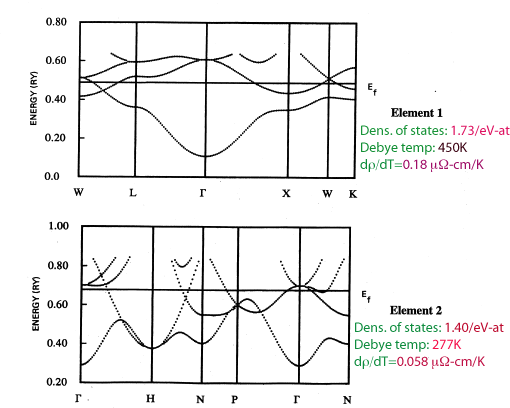BCS theory of superconductivity: the world's largest Madoff scheme?
BCS theory of superconductivity: it is time to question its validity, Physica Scripta 80, 035702 (2009)

Why is BCS theory unable to predict superconducting materials?

The figure below shows a plot of the superconducting critical temperatures of the elements versus the inverse Hall coefficient. Note that superconductors are predominantly found among materials with positive Hall coefficient.Materials with positive Hall coefficient have hole carriers. Materials with negative Hall coefficient have electron carriers. BCS theory doesn't know the difference between electrons and holes, it treats them as equivalent quasiparticles. However as pointed out by Chapnik many years ago, superconductors seem to care a lot about whether the carriers are electrons or holes: if carriers are electrons (negative Hall coefficient) the material, whether element or compound, is not superconducting. BCS theory cannot explain this very general empirical observation.

The theory of hole superconductivity proposes an explanation for why superconductors have to have hole carriers. A simple garage analogy illustrates the basic point qualitatively: single holes have difficulty propagating and pairs of holes can propagate more easily, while for electrons the situation is reversed. This is because holes are more dressed than electrons, and holes undress and turn into electrons when they pair. The theory also implies that the electron-phonon interaction, assumed in BCS theory to be the cause of superconductivity, is in fact irrelevant to superconductivity.

BCS theory is unable to predict new superconductors because it assumes that superconductivity is associated with the electron-phonon interaction. Hence it predicts that superconductivity and high transition temperatures will occur in materials with strong electron-phonon interaction (as evidenced eg by large temperature dependence of the normal state resitivity) , large electronic density of states (as evidenced eg by large normal state heat capacity ) and light ionic mass (as evidenced eg by high Debye temperature). In fact however a statistical analysis of the correlation between these normal state properties and superconductivity in the elements reveals that these properties are NOT strongly associated with superconductivity. Instead, a very strong correlation is found between superconductivity and positive Hall coefficient. The figure below (from Phys. Rev. B 55, 9007 (1997)) shows the degree of association (as measured by the Kolmogoroff-Smirnoff index) between various normal state properties and superconductivity in the elements.The theory of hole superconductivity predicts that superconductivity is associated with hole conduction in the normal state and that high Tc's occur when holes conduct in negatively charged structures, i.e. a lattice of anions. The situations where high Tc's have been found in nature (high Tc cuprates, magnesium diboride, iron arsenides) are in agreement with this expectation.

Chapter test

Can you tell by looking at the band structures below which of these two elements is a superconductor and which is not?Return